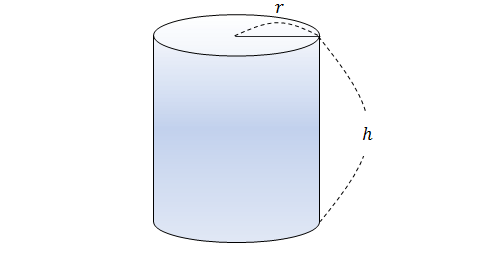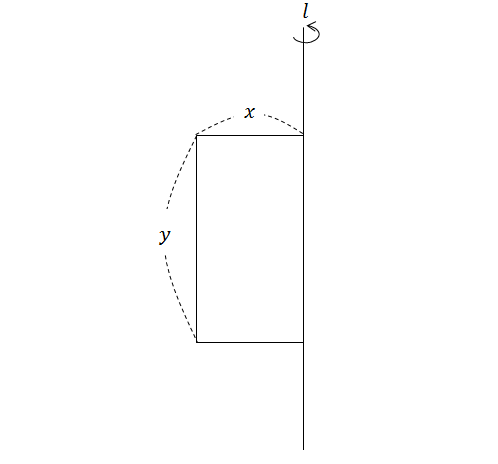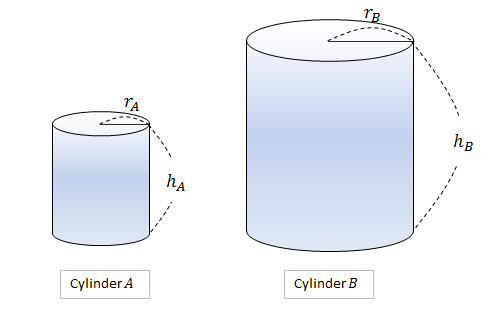Geometry

# Surface Area - Cylinder

What is the surface area of a cylinder with radius $4$ and height $6$?The above diagram shows a cylinder with height $h=9.$ If the surface area of the cylinder is $44\pi,$ what is the radius $r?$

Note: The above figure is not drawn to scale.The above diagram shows a rectangle with $x=4$ and $y=8.$ If the rectangle is rotated $360^\circ$ around line $l,$ what is the surface area of the resulting body?

Note: The above figure is not drawn to scale.The diagram above shows two cylinders with $r_A=2, h_A=4, r_B=3,$ and $h_B=7.$ If $S_A$ and $S_B$ are the surface areas of cylinders A and B, respectively, what is the value of $\frac{S_A}{S_B}?$

Note: The above figures are not drawn to scale.

A cylinder has a total surface area of $136\pi$. If it has a radius of $4$, what is its height?

×